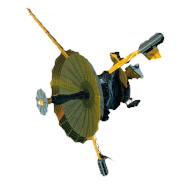GALILEO

## Galileo EPD Handbook

### Chapter 2. EPD Software

EPD SBAND Mission Software Requirements (continued)

Science Background (continued)Table 1. LEMMS Rate Channel Mapping

 Rate Channel Channel Map 1 Channel Map 2 High Low Omni High Low Omni A0 x x A1 x x A2 x x A3 x x A4 x x A5 x x A6 x x A7 x x A8 x x E0 x x E1 x x E2 x x E3 x x F0 x x F1 x x F2 x x F3 x x B0 x x B1 x x B2 x x DC0 x x DC1 x x DC2 x x DC3 x x AS x x BS x x CS x x DS x x EB1 x x EB2 x x FB1 x x FB2 x x Total 4 13 15 7 16 9

Table 2. CMS Rate Channel Mapping

 Rate Channel Channel Map 1 Channel Map 2 High Low Omni High Low Omni TP1 x x TP2 x x TP3 x x TA1 x x TA2 x x TO1 x x TO2 x x TO3 x x TO4 x x TS1 x x TS2 x x TS3 x x TH1 x x TACS x x KTS x x STARTS x x Total 6 9 1 9 7 0